# NCERT Solutions for Class 9 Maths Exercise 12.1## myCBSEguide App

CBSE, NCERT, JEE Main, NEET-UG, NDA, Exam Papers, Question Bank, NCERT Solutions, Exemplars, Revision Notes, Free Videos, MCQ Tests & more.

NCERT solutions for Class 9 Maths Herons Formula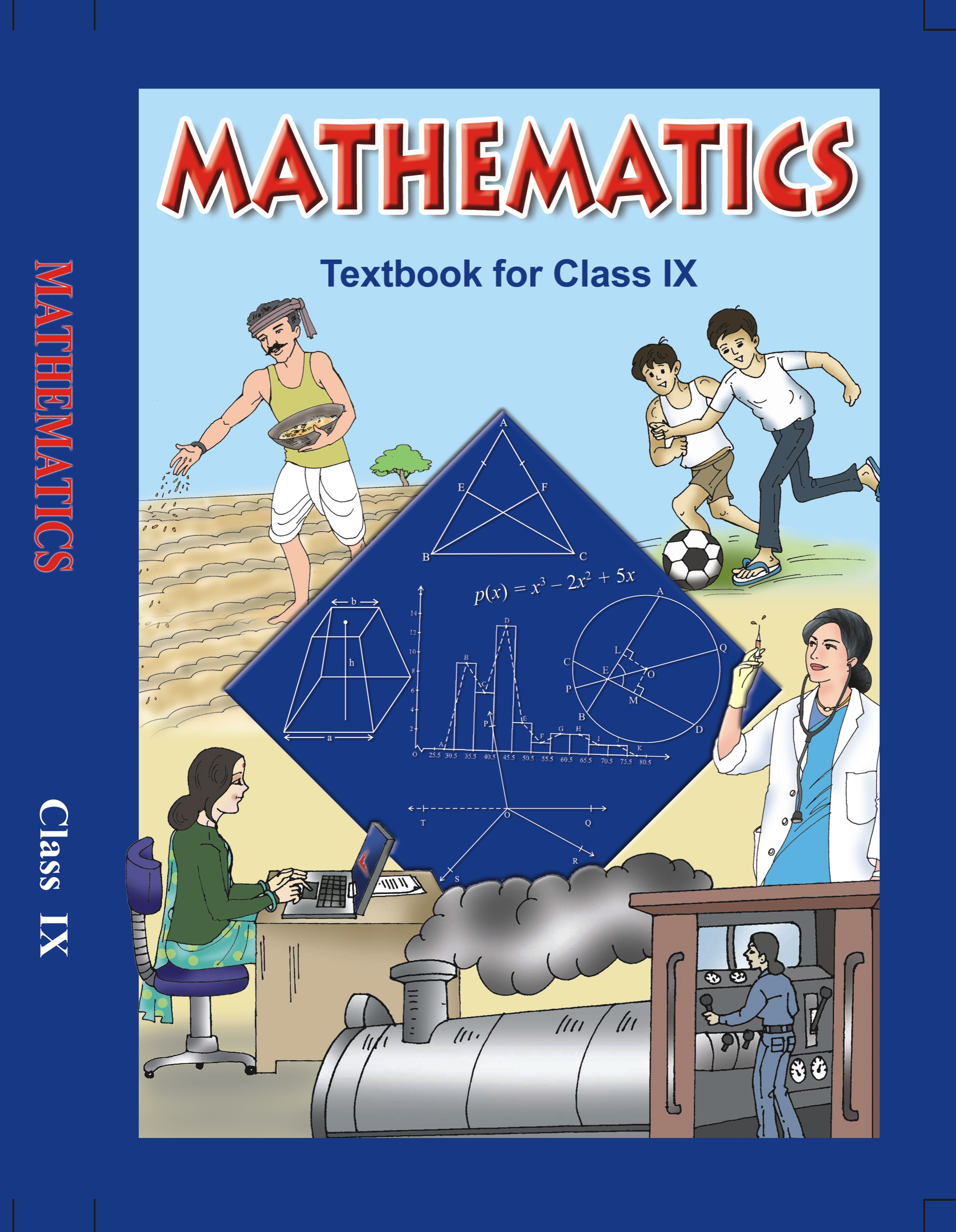## NCERT Solutions for Class 9 Mathematics Herons Formula

1. A traffic signal board, indicating ‘SCHOOLAHEAD’ is an equilateral triangle with side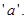Find the area of the signal board, using Heron’s formula. If its perimeter is 180 cm, what will be the area of the signal board?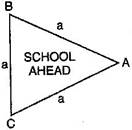Ans. Let the Traffic signal board is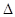ABC.

According to question, Semi-perimeter ofABC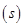=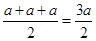Using Heron’s Formula, Area of triangle ABC =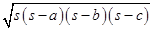=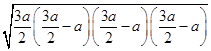=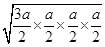=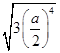=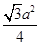Now, Perimeter of this triangle = 180 cm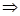Side of triangle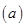=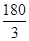= 60 cmSemi-perimeter of this triangle =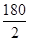= 90 cm

Using Heron’s Formula, Area of this triangle ==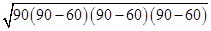=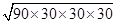=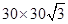=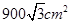2. The triangular side walls of a flyover has been used for advertisements. The sides of the walls are 122 m, 22 m and 120 m (see figure). The advertisement yield an earning of Rs. 5000 per m2 per year. A company hired one of its walls for 3 months, how much rent did it pay?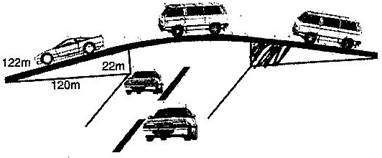Ans. Given: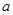= 122 m,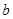= 22 m and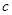= 120 m

Semi-perimeter of triangle=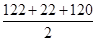=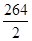= 132 m

Using Heron’s Formula,

Area of triangle ==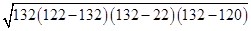=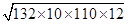=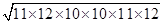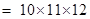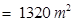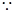Rent for advertisement on wall for 1 year = Rs. 5000 per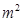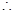Rent for advertisement on wall for 3 months for 1320 m2 =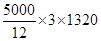= Rs. 1650000

Hence rent paid by company = Rs. 16,50,000

3. There is slide in a park. One of its side walls has been painted in some colour with a message “KEEP THE PARK GREEN AND CLEAN”, (see figure). If the sides of the wall are 15 m, 11 m and 6 m, find the area painted in colour.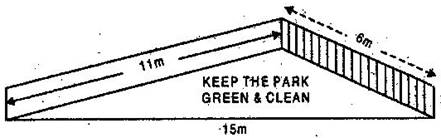Ans. Since, sides of coloured triangular wall are 15 m, 11 m and 6 m.Semi-perimeter of coloured triangular wall =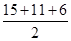=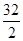= 16 m

Now, Using Heron’s formula,

Area of coloured triangular wall ==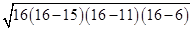=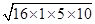=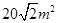Hence area painted in blue colour =4. Find the area of a triangle two sides of which are 18 cm and 10 cm and the perimeter is 42 cm.

Ans. Given:= 18 cm,= 10 cm.

Since Perimeter = 42 cm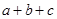=4218 + 10 += 42= 42 – 28 = 14 cmSemi-perimeter of triangle =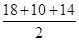= 21 cmArea of triangle ==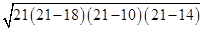=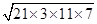=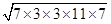=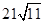= 21 x 3.3 = 69.3 cm2

NCERT Solutions for Class 9 Maths Exercise 12.1

###### 5. Sides of a triangle are in the ratio of 12: 17: 25 and its perimeter is 540 cm. Find its area.

Ans. Let the sides of the triangle be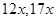and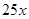.

Therefore,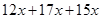= 540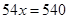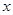= 10The sides are 120 cm, 170 cm and 250 cm.

Semi-perimeter of triangle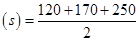= 270 cm

Now, Area of triangle ==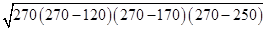=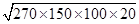=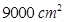NCERT Solutions for Class 9 Maths Exercise 12.1

###### 6. An isosceles triangle has perimeter 30 cm and each of the equal sides is 12 cm. Find the area of the triangle.

Ans. Given:= 12 cm,= 12 cm

Since Perimeter = 30 cm=3012 + 12 += 30= 30 – 24 = 6 cmSemi-perimeter of triangle =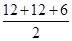= 15 cmArea of triangle ==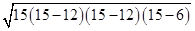=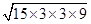=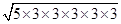=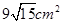## NCERT Solutions for Class 9 Maths Exercise 12.1

NCERT Solutions for Class 9 Maths PDF (Download) Free from myCBSEguide app and myCBSEguide website. Ncert solution class 9 Maths includes text book solutions from Mathematics Book. NCERT Solutions for CBSE Class 9 Maths have total 15 chapters. 9 Maths NCERT Solutions in PDF for free Download on our website. Ncert Maths class 9 solutions PDF and Maths ncert class 9 PDF solutions with latest modifications and as per the latest CBSE syllabus are only available in myCBSEguide.

## CBSE app for Class 9

To download NCERT Solutions for Class 9 Maths, Computer Science, Home Science,Hindi ,English, Social Science do check myCBSEguide app or website. myCBSEguide provides sample papers with solution, test papers for chapter-wise practice, NCERT solutions, NCERT Exemplar solutions, quick revision notes for ready reference, CBSE guess papers and CBSE important question papers. Sample Paper all are made available through the best app for CBSE students and myCBSEguide website.### 29 thoughts on “NCERT Solutions for Class 9 Maths Exercise 12.1”

1. Thankyou for this….

2. Thank you very much.

3. Thank you it is very good

4. Thank you it is very good

5. Thank u i was juz doin the prob but i didnt get de answer so i juz flipped into these .thank u .its better understanding

6. Thank u for simple methods

7. Thank very god

8. Thanku it is very good

9. Thank you this help me more

10. Thanks realy it is very good

11. Nice explanation

12. thanks for math solution

13. Thanku very thanku
Nice very nice

14. THANK YOU, it is very nice, I LIKE IT.

15. Thanx for this

16. Thankew

17. Thank you very much

I am very thank for this

18. It’s very well explained answer. I wish this my cbse Guide. Com must be in top

20. Thanks ???

21. Keep it up.
Nice

22. I was having some problems so I fleb, this helps me better understanding (tq so much)

23. It’s good carry on (tq 4 helping me with better understanding)

24. Well done I appreciate this work great!